Open In App
Related Articles
• RD Sharma Class 9 Solutions

# Class 9 RD Sharma Solution – Chapter 10 Congruent Triangles- Exercise 10.5

### Question 1. ABC is a triangle and D is the mid-point of BC. The perpendiculars from D to AB and AC are equal. Prove that the triangle is isosceles.

Solution: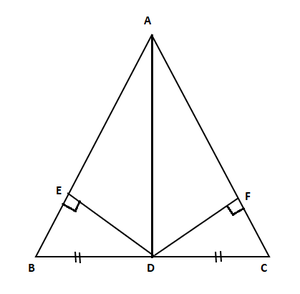Given: D is the mid-point of BC so, BD = DC, ED = FD, and ED ⊥ AB, FD ⊥ AC, so ED = FD

Prove: ΔABC is an isosceles triangle

In ΔBDE and ΔCDF

ED = FD [Given]

BD = DC [D is mid-point]

∠BED = ∠CFD = 90°

By RHS congruence criterion

ΔBDE ≅ ΔCDF

So, now by C.P.C.T

BE = CF … (i)

Now, in △AED and △AFD

ED = FD [Given]

∠AED = ∠AFD = 90°

By RHS congruence criterion

△AED ≅ △AFD

So, now by C.P.C.T

So, EA = FA … (ii)

Now by adding equation (i) and (ii), we get

BE + EA = CF + FA

AB = AC

So, ΔABC is an isosceles triangle because two sides of the triangle are equal.

Hence proved

### Question 2. ABC is a triangle in which BE and CF are, respectively, the perpendiculars to the sides AC and AB. If BE = CF, prove that ΔABC is isosceles.

Solution: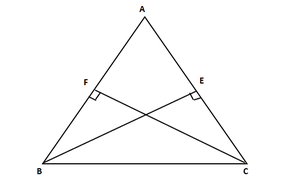Given: BE ⊥ AC, CF ⊥ AS, BE = CF.

To prove: ΔABC is isosceles

In ΔBCF and ΔCBE,

∠BFC = CEB = 90° [Given]

BC = CB [Common side]

And CF = BE [Given]

By RHS congruence criterion

ΔBFC ≅ ΔCEB

So, now by C.P.C.T

∠FBC = ∠EBC

∠ABC = ∠ACB

and AC = AB [Because opposite sides to equal angles are equal]

So, ΔABC is isosceles

Hence proved

### Question 3. If perpendiculars from any point within an angle on its arms are congruent. Prove that it lies on the bisector of that angle.

Solution: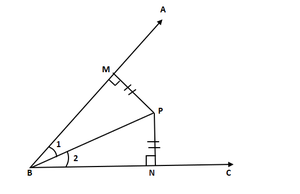Let us consider ∠ABC and BP is an arm within∠ABC

So now draw perpendicular from point P on arm BA and BC, i.e., PN and PM

Prove: BP is the angular bisector of ∠ABC.

In ΔBPM and ΔBPN

∠BMP = ∠BNP = 90° [Given]

MP = NP [Given]

BP = BP [Common side]

So, by RHS congruence criterion

ΔBPM ≅ ΔBPN

So, by C.P.C.T

∠MBP = ∠NBP

and BP is the angular bisector of ∠ABC.

Hence proved

### Question 4. In figure, AD ⊥ CD and CB ⊥ CD. If AQ = BP and DP = CQ, prove that ∠DAQ = ∠CBP.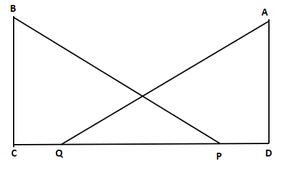Solution:

Given that AD ⊥ CD, CB ⊥ CD, AQ = BP and DP = CQ,

Prove:∠DAQ = ∠CBP

We have DP = CQ

So by adding PQ on both sides, we get

DP + PQ = CQ + PQ

DQ = CP … (i)

In ΔDAQ and ΔCBP

We have

∠ADQ = ∠BCP = 90° [Given]

And DQ = PC [From (i)]

So, by RHS congruence criterion

ΔDAQ ≅ ΔCBP

So, by C.P.C.T

∠DAQ = ∠CBP

Hence proved

### Question 5. ABCD is a square, X and Y are points on sides AD and BC respectively such that AY = BX. Prove that BY = AX and ∠BAY = ∠ABX.

Solution: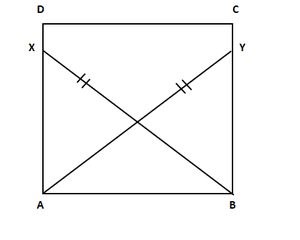In ABCD square,

X and Y are points on sides AD and BC

So, AY = BX.

To prove: BY = AX and ∠BAY = ∠ABX

Now, join Band X, A and Y

So,

∠DAB = ∠CBA = 90° [Given ABCD is a square]

Also, ∠XAB = ∠YAB = 90°

In ΔXAB and ΔYBA

∠XAB = ∠YBA = 90° [given]

AB = BA [Common side]

So, by RHS congruence criterion

ΔXAB ≅ ΔYBA

So, by C.P.C.T

BY = AX

∠BAY = ∠ABX

Hence proved

### Question 6. Which of the following statements are true (T) and which are false (F):

(i) Sides opposite to equal angles of a triangle may be unequal.

(ii) Angles opposite to equal sides of a triangle are equal

(iii) The measure of each angle of an equilateral triangle is 60

(iv) If the altitude from one vertex of a triangle bisects the opposite side, then the triangle may be isosceles.

(v) The bisectors of two equal angles of a triangle are equal.

(vi) If the bisector of the vertical angle of a triangle bisects the base, then the triangle may be isosceles.

(vii) The two altitudes corresponding to two equal sides of a triangle need not be equal.

(viii) If any two sides of a right triangle are respectively equal to two sides of other right triangle, then the two triangles are congruent.

(ix) Two right-angled triangles are congruent if the hypotenuse and a side of one triangle are respectively equal to the hypotenuse and a side of the other triangle.

Solution:

(i) False

(ii) True

(iii) True

(iv) False

(v) True

(vi) False

(vii) False

(viii) False

(ix) True

### Question 7. Fill the blanks In the following so that each of the following statements is true.

(i) Sides opposite to equal angles of a triangle are ___

(ii) Angle opposite to equal sides of a triangle are ___

(iii) In an equilateral triangle all angles are ___

(iv) In ΔABC, if ∠A = ∠C, then AB =

(v) If altitudes CE and BF of a triangle ABC are equal, then AB  ___

(vi) In an isosceles triangle ABC with AB = AC, if BD and CE are its altitudes, then BD is ___ CE.

(vii) In right triangles ABC and DEF, if hypotenuse AB = EF and side AC = DE, then ΔABC ≅ Δ ___

Solution:

(i) Equal

(ii) Equal

(iii) Equal

(iv) AB = BC

(v) AB = AC

(vi) BD is equal to CE

(vii) ΔABC ≅ ΔEFD.